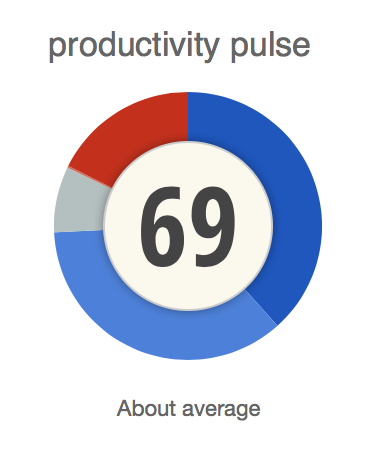# How is my Productivity Pulse calculated?

The productivity pulse is a benchmark score of your productivity from 0 - 100.Every activity in RescueTime has a "productivity score" associated with it. Usually, this is inherited from the category that the activity resides in, but the user can directly override the score

Those scores, along with the time spent in different activities, are used to calculate your "Productivity Pulse."

If you spend more time in activities that you have marked as productive, the pulse will rise. If you spend more time in activities marked as distracting, the number will shrink.

Think of it as a simple baseline that will give you an at-a-glance idea of how your time is being spent.

The math behind the productivity pulse works like this:

```(((vd * 0) + (d * 1) + (n * 2) + (p * 3) + (vp * 4)) / (total * 4)) * 100
```

Where :

```vd = very distracting time
```
```d = distracting time
```
```n = neutral time
```
```p = productive time
```
```vp = productive time
```
```total = your total time logged for the time period
```

To put this another way:

• If 100% of your time was logged as "very distracting time", your productivity pulse for that period would be zero
• If 100% of your time was logged as "distracting time", your productivity pulse for that period would be 25
• If 100% of your time was logged as "productive time", your productivity pulse for that period would be 75
• If 100% of your time was logged as "very productive time", your productivity pulse for that period would be 100
• If 50% of your time was logged as "very distracting time", and 50% was logged as "very productive time", your productivity pulse for that period would be 50